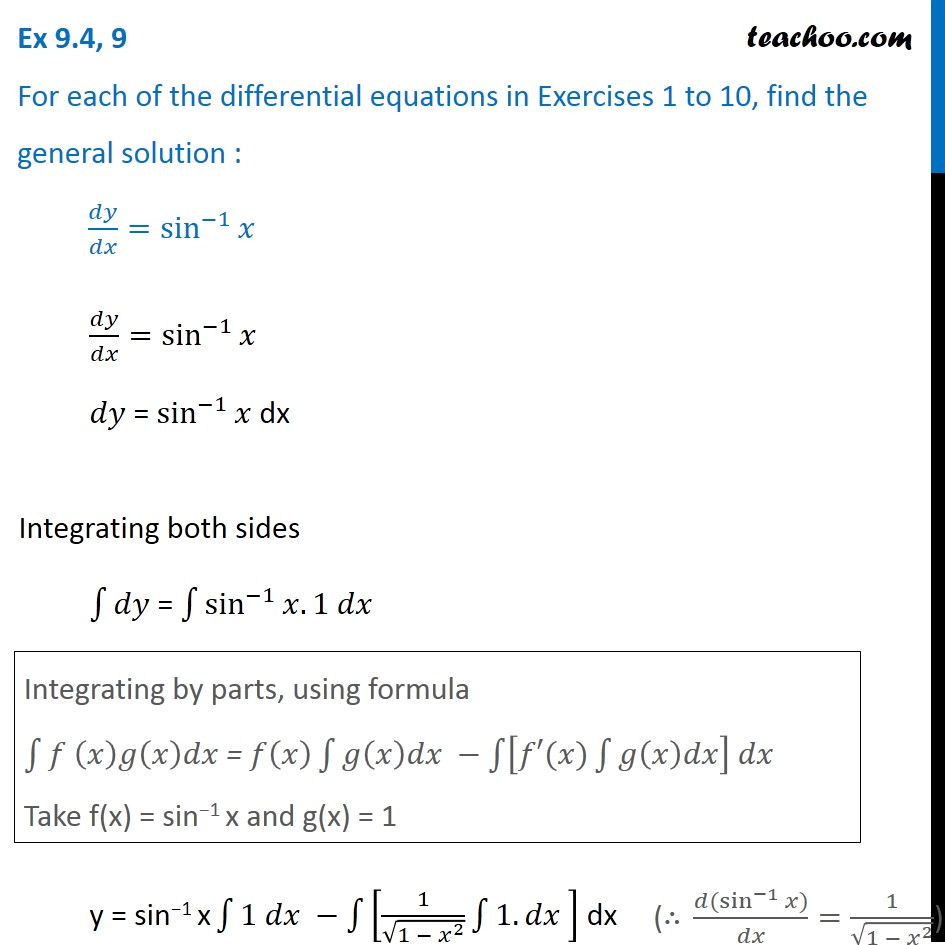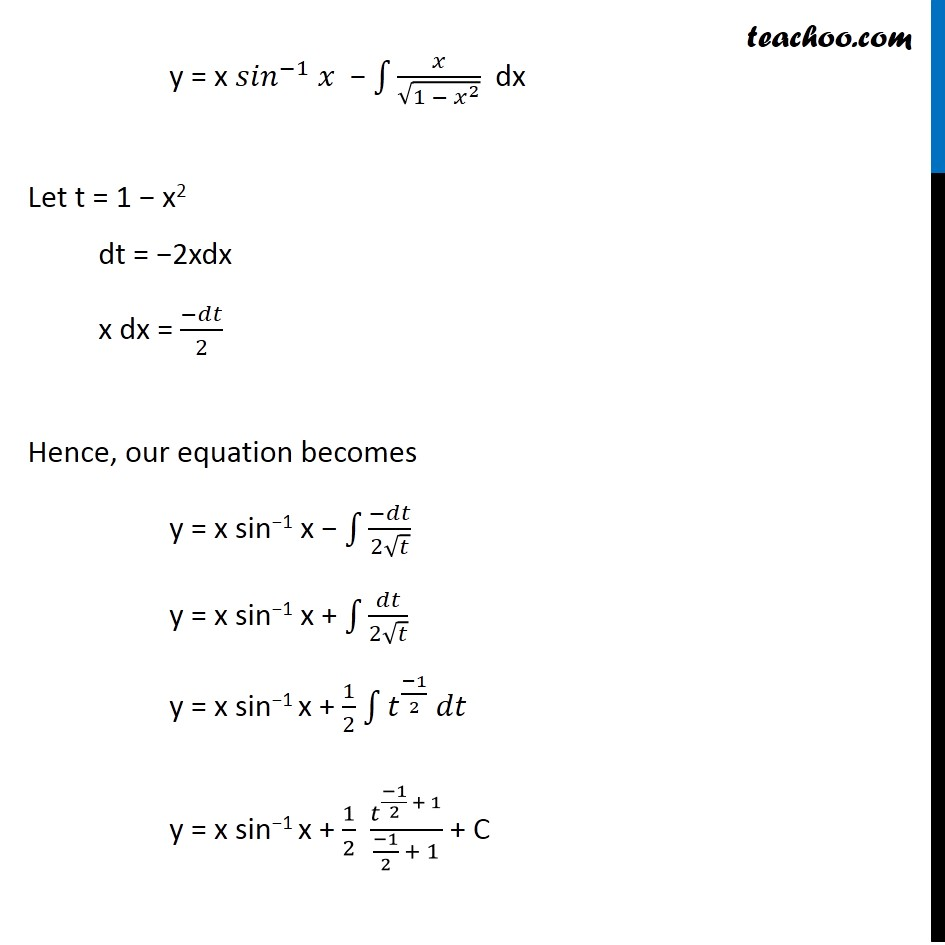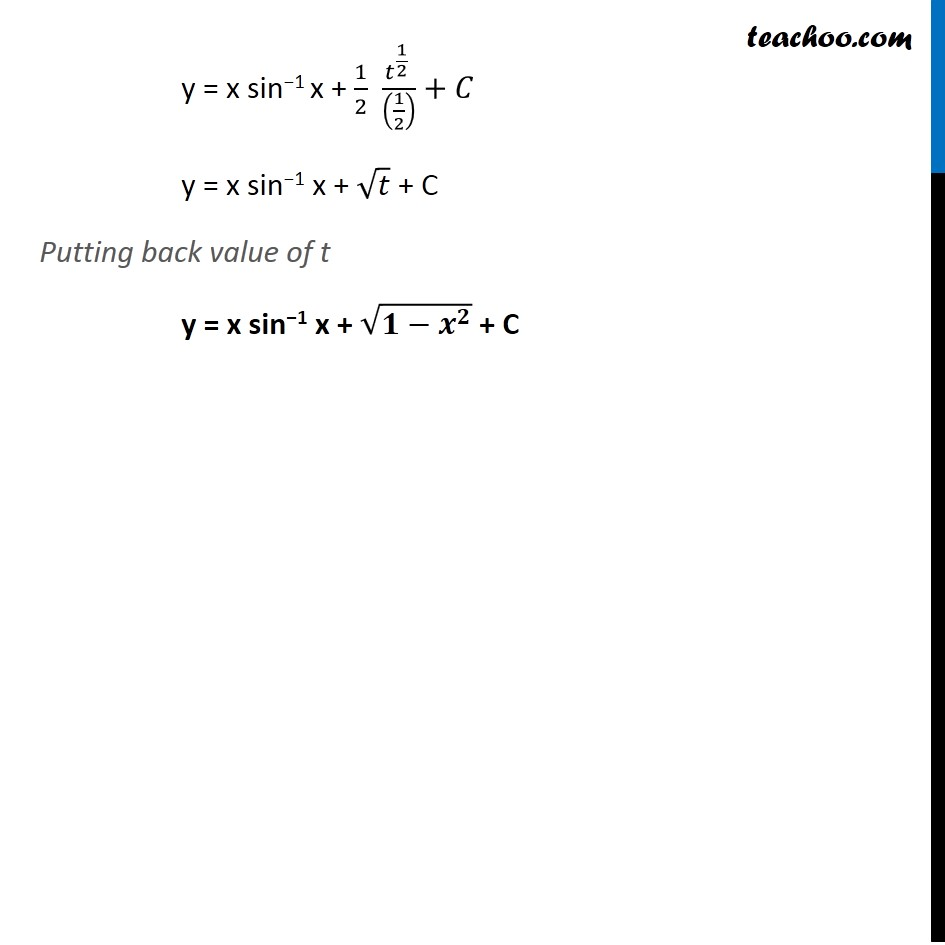Ex 9.4

Chapter 9 Class 12 Differential Equations
Serial order wiseGet live Maths 1-on-1 Classs - Class 6 to 12

### Transcript

Ex 9.4, 9 For each of the differential equations in Exercises 1 to 10, find the general solution : 𝑑𝑦/𝑑𝑥=sin^(−1)⁡𝑥 𝑑𝑦/𝑑𝑥=sin^(−1)⁡𝑥 𝑑𝑦 = sin^(−1)⁡𝑥 dx Integrating both sides ∫1▒〖𝑑𝑦 〗= ∫1▒〖sin^(−1)⁡〖𝑥.1 𝑑𝑥〗 〗 y = sin−1 x ∫1▒〖1 𝑑𝑥 −∫1▒[1/√(1 − 𝑥^2 ) ∫1▒〖1.𝑑𝑥 〗] 〗 dx Integrating by parts, using formula ∫1▒〖𝑓 (𝑥)𝑔(𝑥)𝑑𝑥 〗= 𝑓(𝑥) ∫1▒〖𝑔(𝑥)𝑑𝑥 −∫1▒〖[𝑓′(𝑥)∫1▒𝑔(𝑥)𝑑𝑥] 𝑑𝑥 〗 〗 Take f(x) = sin−1 x and g(x) = 1 ("∴" (𝑑〖(sin〗^(−1) 𝑥))/𝑑𝑥=1/√(1 − 𝑥^2 )) y = x 〖𝑠𝑖𝑛〗^(−1) 𝑥 − ∫1▒𝑥/√(1 − 𝑥^2 ) dx Let t = 1 − x2 dt = −2xdx x dx = (−𝑑𝑡)/2 Hence, our equation becomes y = x sin−1 x − ∫1▒(−𝑑𝑡)/(2√𝑡) y = x sin−1 x + ∫1▒𝑑𝑡/(2√𝑡) y = x sin−1 x + 1/2 ∫1▒〖𝑡^((−1)/2) 𝑑𝑡〗 y = x sin−1 x + 1/2 𝑡^((−1)/2 + 1)/((−1)/2 + 1) + C y = x sin−1 x + 1/2 (𝑡^(1/2) )/((1/2) )+𝐶 y = x sin−1 x + √𝑡 + C Putting back value of t y = x sin−1 x + √(𝟏−𝒙^𝟐 ) + C